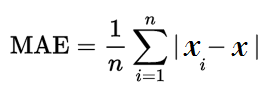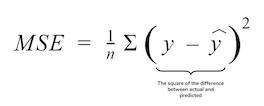# Regression Problems Performance Measurement

Hey - Nick here! This page is a free excerpt from my new eBook Pragmatic Machine Learning, which teaches you real-world machine learning techniques by guiding you through 9 projects.

In the last lesson of this course, we discussed metrics used to assess the performance of classification problems in machine learning.

Let's continue our discussion of performance metrics by learning about performance metrics used in regression problems.

## What is a Regression Problem?

Before you can learn about how to measure the performance of a machine learning regression problem, you should first understand what these types of problems are.

Regression problems are those that attempt to predict continuous values. For comparison, classification problems attempt to predict categorical values.

## Examples of Regression Problems

An example can be helpful to understand how we typically structure regression problems.

Imagine that you have a group of students in a machine learning class. You'd like to predict their final grade in the class (in percent) using already-known metrics like age, attendence, and previous test scores. Since their grade (in percent) is continuous - meaning it can take on any value in its range from 0 to 100 - this is a regression problem.

This example is useful because if we change it slightly, it becomes a classification problem. More specifically, if we use the letter-grade scheme, we group students grades into five categories: A, B, C, D, and F.

Since there are distinct categories that appear using this scheme, it becomes a classification problem.

Now that you understand how to structure regression problems, let's learn how to measure their performance.

## How to Measure Regression Performance

There are three main evaluation metrics used for regression problems:

1. Mean Absolute Error
2. Mean Squared Error
3. Root Mean Squared Error

We will discuss each metric one-by-one in this tutorial.

## Regression Performance Metric #1: Mean Absolute Error

Mean absolute error is likely the easiest performance metric to understand. We calculate this score exactly as you'd expect - by taking the mean of the absolute value of the algorithm's errors.

Here is the formal equation for mean absolute error:Let's use our earlier example of predicting students final grades to see how to calculate mean absolute error. Imagine you predicted a student's grade to be 95 and they actually scored 91. Their absolute error is 4 (the absolute value of 91 minus 95). You calculate mean absolute error by taking the average of this figure across the entire class.

Mean absolute error has the advantage of being easy to understand. It also has a significant downside.

Specifically, mean absolute error does not punish large errors. If your data set has a few significant outliers, then this will have an smaller-than-necessary impact on the mean absolute error of your algorithm.

## Regression Performance Metric #2: Mean Squared Error

Mean squared error is similar to mean absolute error except that instead of taking the absolute value of the difference, you square it instead.

Here is the formal equation for mean squared error:The mean squared error is more popular than the mean absolute error because it increases the importance of large errors. As you can imagine, squaring a large error has a more notable effect on its value than squaring a small error.

Mean squared error introduces another problem, however. Squaring the error values also squares the units that their measured in. If your predicted value is in miles, then its root mean squared error value will be in square miles.

To fix this, we use the root mean squared error, which we talk about next.

## Regression Performance Metric #3: Root Mean Squared Error

You can calculate the root mean squared error by taking the differences of each data point's actual value and its predicted value, then squaring and square-rooting its value.

Here is the formal equation for root mean squared error:Root mean squared error is the most popular performance metric for machine learning regression problems.

## Final Thoughts

In this tutorial, you learned how to measure the performance of machine learning regression algorithms.

Here is a brief summary of what you learned in this lesson:

• How regression problems deal with continuous data and classification problems deal with categorical data
• An example of a machine learning regression problem
• The three most important performance metrics for machine learning regression problems (and the problems associated with each metric)